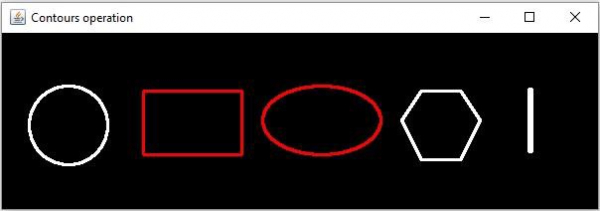# How to find the area of an image contour Java OpenCV library?

Contours are nothing but the line joining all the points along the boundary of a particular shape. Using this you can −

• Find the shape of an object.

• Calculate the area of an object.

• Detect an object.

• Recognize an object.

You can find the contours of various shapes, objects in an image using the findContours() method. In the same way you can draw

You can also find the area of the shapes in the given input images. To do so you need to invoke the contourArea() method of the Imgproc class. This method accepts the contour of a particular shape, finds and returns its area.

## Example

Following java example finds the area of each shape/object in the given image, draw the outlines of the shapes with an area less great than 5000 in red and the remaining in white.

import java.util.ArrayList;
import java.util.List;
import org.opencv.core.Core;
import org.opencv.core.CvType;
import org.opencv.core.Mat;
import org.opencv.core.MatOfPoint;
import org.opencv.core.Point;
import org.opencv.core.Scalar;
import org.opencv.highgui.HighGui;
import org.opencv.imgcodecs.Imgcodecs;
import org.opencv.imgproc.Imgproc;
public class FindContourArea {
public static void main(String args[]) throws Exception {
//Reading the contents of the image
String file ="D:\Images\javafx_graphical.jpg";
//Converting the source image to binary
Mat gray = new Mat(src.rows(), src.cols(), src.type());
Imgproc.cvtColor(src, gray, Imgproc.COLOR_BGR2GRAY);
Mat binary = new Mat(src.rows(), src.cols(), src.type(), new Scalar(0));
Imgproc.threshold(gray, binary, 100, 255, Imgproc.THRESH_BINARY_INV);
//Finding Contours
List<MatOfPoint> contours = new ArrayList<>();
Mat hierarchey = new Mat();
Imgproc.findContours(binary, contours, hierarchey, Imgproc.RETR_TREE,
Imgproc.CHAIN_APPROX_SIMPLE);
Mat draw = Mat.zeros(src.size(), CvType.CV_8UC3);
for (int i = 0; i < contours.size(); i++) {
Scalar color = new Scalar(0, 0, 255);
//Calculating the area
double cont_area = Imgproc.contourArea(contours.get(i));
System.out.println(cont_area);
if(cont_area>5000.0){
Imgproc.drawContours(draw, contours, i, color, 2,
Imgproc.LINE_8, hierarchey, 2, new Point() ) ;
} else {
color = new Scalar(255, 255, 255);
Imgproc.drawContours(draw, contours, i, color, 2, Imgproc.LINE_8,
hierarchey, 2, new Point() ) ;
}
}
HighGui.imshow("Contours operation", draw);
HighGui.waitKey();
}
}

Input Image

## Output

4091.0
6336.0
189.0
6439.0
4903.0

In addition to the above output, the above program generates the following window −